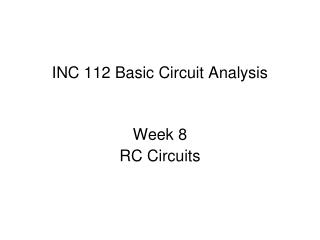DownloadDownload PresentationINC 112 Basic Circuit Analysis

# INC 112 Basic Circuit Analysis

Télécharger la présentation## INC 112 Basic Circuit Analysis

- - - - - - - - - - - - - - - - - - - - - - - - - - - E N D - - - - - - - - - - - - - - - - - - - - - - - - - - -
##### Presentation Transcript

1. INC 112 Basic Circuit Analysis Week 8 RC Circuits

2. RC Circuits • The response of RC circuits can be categorized into two parts: • Transient Response • Forced Response • Transient response comes from the dynamic of R,C. • Forced response comes from the voltage source.

3. Source-Free RC Circuits Capacitor has some energy stored so that The initial voltage at t=0 is V0 Initial condition Find i(t) from R, C

4. Compare with the solution of RL circuits. The solution of RC circuits can be obtained with the same method. Source-free RC Source-free RL

5. v(t) V0 t i(t) V0/R or t

6. Time Constant The product RC is time constant for RC circuits Unit: second

7. Forced RC Circuits C has an initial voltage of 0 from Use KVL, we got

8. From Differentiate both sides Solve first-order differential equation Where I0 is the initial current of the circuit

9. Natural Response Force Response C has an initial voltage = 0, But from KVL, therefore,and So,

10. vC(t) i(t) V/R V t t vR(t) Note: Capacitor’s voltage cannot abruptly change V t

11. How to Solve Problems? (RC) • Start by finding the voltage of the capacitor first • Assume the response that we want to find is in form of • Find the time constant τ (may use Thevenin’s) • Solve for k1, k2 using initial conditions and • status at the stable point • From the voltage, find other values that the problem ask • using KCL, KVL

12. Example Switch open for a long time before t=0, find and sketch i(t) First, we start by finding vc(t) The initial condition of C is vc(0) = 1V The stable conditionof C is vc(∞) = 3V

13. Assume vc(t) in form of Find the time constant after t=0 by Thevenin’s, viewing C as a load Therefore, the time constant is

14. Find k1, k2 using vc(0) = 1, vc(∞) = 3 At t=0, vc(0) = 1 V At t = ∞, vc(∞) = 3 V Therefore, k1=3, k2 = -2 We can find i(t) by using Ohm’s law on the resistor

15. i(t) 4A 2A t

16. Example The switch was opened for a long time before t=0, Find i(t) Start with vc(t) The initial condition of C is vc(0) = 5V The final stable conditionof C comes from voltage divider, which is vc(∞) = 5*(1/1+0.5) = 3.33V

17. Assume vc(t) in form of Find the time constant after t=0 by Thevenin’s, viewing C as a load Therefore, the time constant is

18. Find k1, k2 using vc(0) = 5, vc(∞) = 3.33 At t=0, vc(0) = 5 V At t = ∞, vc(∞) = 3.33 V Therefore, k1=3.33, k2 = 1.66 We can find i(t) by using Ohm’s law on the resistor

19. i(t) 1.66mA t vc(t) 5V 3.33V t